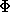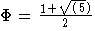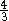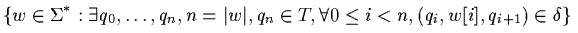Next: Brute Force algorithm Up: ESMAJ Prev: Contents

# Introduction

Introduction

String-matching is a very important subject in the wider domain of text processing. String-matching algorithms are basic components used in implementations of practical softwares existing under most operating systems. Moreover, they emphasize programming methods that serve as paradigms in other fields of computer science (system or software design). Finally, they also play an important role in theoretical computer science by providing challenging problems.

Although data are memorized in various ways, text remains the main form to exchange information. This is particularly evident in literature or linguistics where data are composed of huge corpus and dictionaries. This apply as well to computer science where a large amount of data are stored in linear files. And this is also the case, for instance, in molecular biology because biological molecules can often be approximated as sequences of nucleotides or amino acids. Furthermore, the quantity of available data in these fields tend to double every eighteen months. This is the reason why algorithms should be efficient even if the speed and capacity of storage of computers increase regularly.

String-matching consists in finding one, or more generally, all the occurrences of a string (more generally called a pattern) in a text. All the algorithms in this book output all occurrences of the pattern in the text. The pattern is denoted by x=x[0 .. m-1]; its length is equal to m. The text is denoted by y=y[0 .. n-1]; its length is equal to n. Both strings are build over a finite set of character called an alphabet denoted bywith size is equal to.

Applications require two kinds of solution depending on which string, the pattern or the text, is given first. Algorithms based on the use of automata or combinatorial properties of strings are commonly implemented to preprocess the pattern and solve the first kind of problem. The notion of indexes realized by trees or automata is used in the second kind of solutions. This book will only investigate algorithms of the first kind.

String-matching algorithms of the present book work as follows. They scan the text with the help of a window which size is generally equal to m. They first align the left ends of the window and the text, then compare the characters of the window with the characters of the pattern - this specific work is called an attempt - and after a whole match of the pattern or after a mismatch they shift the window to the right. They repeat the same procedure again until the right end of the window goes beyond the right end of the text. This mechanism is usually called the sliding window mechanism. We associate each attempt with the position j in the text when the window is positioned on y[j .. j+m-1].

The Brute Force algorithm locates all occurrences of x in y in time O(mn). The many improvements of the brute force method can be classified depending on the order they performed the comparisons between pattern characters and text characters et each attempt. Four categories arise: the most natural way to perform the comparisons is from left to right, which is the reading direction; performing the comparisons from right to left generally leads to the best algorithms in practice; the best theoretical bounds are reached when comparisons are done in a specific order; finally there exist some algorithms for which the order in which the comparisons are done is not relevant (such is the brute force algorithm).

From left to right

Hashing provides a simple method that avoids the quadratic number of character comparisons in most practical situations, and that runs in linear time under reasonable probabilistic assumptions. It has been introduced by Harrison and later fully analyzed by Karp and Rabin.

Assuming that the pattern length is no longer than the memory-word size of the machine, the Shift Or algorithm is an efficient algorithm to solve the exact string-matching problem and it adapts easily to a wide range of approximate string-matching problems.

The first linear-time string-matching algorithm is from Morris and Pratt. It has been improved by Knuth, Morris and Pratt. The search behaves like a recognition process by automaton, and a character of the text is compared to a character of the pattern no more than log(m+1) (is the golden ratio). Hancart proved that this delay of a related algorithm discovered by Simon makes no more than 1+log2m comparisons per text character. Those three algorithms perform at most 2n-1 text character comparisons in the worst case.

The search with a Deterministic Finite Automaton performs exactly n text character inspections but it requires an extra space in O(m). The Forward Dawg Matching algorithm performs exactly the same number of text character inspections using the suffix automaton of the pattern.

The Apostolico-Crochemore algorithm is a simple algorithm which performsn text character comparisons in the worst case.

The Not So Naive algorithm is a very simple algorithm with a quadratic worst case time complexity but it requires a preprocessing phase in constant time and space and is slightly sub-linear in the average case.

From right to left

The Boyer-Moore algorithm is considered as the most efficient string-matching algorithm in usual applications. A simplified version of it (or the entire algorithm) is often implemented in text editors for the "search" and "substitute" commands. Cole proved that the maximum number of character comparisons is tightly bounded by 3n after the preprocessing for non-periodic patterns. It has a quadratic worst case time for periodic patterns.

Several variants of the Boyer-Moore algorithm avoid its quadratic behaviour. The most efficient solutions in term of number of symbol comparisons have been designed by Apostolico and Giancarlo, Crochemore et alii (Turbo BM), and Colussi (Reverse Colussi).

Empirical results show that the variations of Boyer and Moore's algorithm designed by Sunday (Quick Search) and an algorithm based on the suffix automaton by Crochemore et alii (Reverse Factor and Turbo Reverse Factor) are the most efficient in practice.

The Zhu and Takaoka and Berry-Ravindran algorithms are variants of the Boyer-Moore algorithm which require an extra space in O(2).

In a specific order

The two first linear optimal space string-matching algorithms are due to Galil-Seiferas and Crochemore-Perrin (Two Way algorithm). They partition the pattern in two parts, they first search for the right part of the pattern from left to right and then if no mismatch occurs they search for the left part.

The algorithms of Colussi and Galil-Giancarlo partition the set of pattern positions into two subsets. They first search for the pattern characters which positions are in the first subset from left to right and then if no mismatch occurs they search for the remaining characters from left to right. The Colussi algorithm is an improvement over the Knuth-Morris-Pratt algorithm and performs at mostn text character comparisons in the worst case. The Galil-Giancarlo algorithm improves the Colussi algorithm in one special case which enables it to perform at mostn text character comparisons in the worst case.

Sunday's Optimal Mismatch and Maximal Shift algorithms sort the pattern positions according their character frequency and their leading shift respectively.

Skip Search, KMP Skip Search and Alpha Skip Search algorithms by Charras (et alii) use buckets to determine starting positions on the pattern in the text.

In any order

The Horspool algorithm is a variant of the Boyer-Moore algorithm, it uses one of his shift function and the order in which the text character comparisons are performed is irrelevant. This is also true for all the other variants such as the Quick Search of Sunday, Tuned Boyer-Moore of Hume and Sunday, the Smith algorithm and the Raita algorithm.

Definitions

We will consider practical searches. We will assume that the alphabet is the set of ASCII codes or any subset of it. The algorithms are presented in C programming language, thus for a word w of lengththe characters are w, ... ,w[-1] and w[] contained the special end character (null character) that cannot occur anywhere within any word but in the end. Both words the pattern and the text reside in main memory.

Let us introduce some definitions.

A word u is a prefix of a word w is there exists a word v (possibly empty) such that w=uv.

A word v is a suffix of a word w is there exists a word u (possibly empty) such that w=uv.

A word z is a substring or a subword or a factor of a word w is there exist two words u and v (possibly empty) such that w=uzv.

An integer p is a period of a word w if for i, 0i < m-p, w[i]=w[i+p]. The smallest period of w is called the period, it is denoted by per(w).

A word w of lengthis periodic if the length of his smallest period is smaller or equal to/2, otherwise it is non-periodic.

A word w is basic if it cannot be written as a power of another word: there exist no word z and no integer k such that w=zk.

A word z is a border of a word w if there exist two words u and v such that w=uz=zv, z is both a prefix and a suffix of w. Note that in this case |u|=|v| is a period of w.

The reverse of a word w of lengthdenoted by wR is the mirror image of w; wR=w[-1]w[-2] ... ww.

A Deterministic Finite Automaton (DFA) A is a quadruple (Q, q0, T, E) where:Q is a finite set of states;q0 in Q is the initial state;T, subset of Q, is the set of terminal states;E, subset of (Q..Q), is the set of transitions.

The language L(A) defined by A is the following set:For each exact string-matching algorithm presented in the present book we first give its main features, then we explained how it works before giving its C code. After that we show its behaviour on a typical example where x=GCAGAGAG and y=GCATCGCAGAGAGTATACAGTACG. Finally we give a list of references where the reader will find more detailed presentations and proofs of the algorithm. At each attempt, matches are materialized in light gray while mismatches are shown in dark gray. A number indicates the order in which the character comparisons are done except for the algorithms using automata where the number represents the state reached after the character inspection.

Implementation

In this book, we use C strings and assume that a string of length m contains a (m+1)-th symbol (usually assigned with the value '\0'). Otherwise some algorithm implementations may crashed when accessing to a position just beyond the actual last character of a string. (Thank you to David B. Trout from Software Development Laboratories for pointing this particular point).

In this book, we will use classical tools. One of them is a linked list of integer. It will be defined in C as follows:

```  struct _cell{
int element;
struct _cell *next;
};

typedef struct _cell *List;
```

Another important structures are automata and specifically suffix automata (see chapter 22). Basically automata are directed graphs. We will use the following interface to manipulate automata:

```  Graph newGraph(int v, int e);
Graph newAutomaton(int v, int e);
Graph newSuffixAutomaton(int v, int e);
int newVertex(Graph g);
int getInitial(Graph g);
boolean isTerminal(Graph g, int v);
void setTerminal(Graph g, int v);
int getTarget(Graph g, int v, unsigned char c);
void setTarget(Graph g, int v, unsigned char c, int t);
void setSuffixLink(Graph g, int v, int s);
int getLength(Graph g, int v);
void setLength(Graph g, int v, int ell);
int getPosition(Graph g, int v);
void setPosition(Graph g, int v, int p);
int getShift(Graph g, int v, unsigned char c);
void setShift(Graph g, int v, unsigned char c, int s);
void copyVertex(Graph g, int target, int source);
```

A possible implementation of this interface follows.

```  struct _graph {
int vertexNumber,
edgeNumber,
vertexCounter,
initial,
*terminal,
*target,
*length,
*position,
*shift;
};

typedef struct _graph *Graph;
typedef int boolean;

#define UNDEFINED -1

/* returns a new data structure for
a graph with v vertices and e edges */
Graph newGraph(int v, int e) {
Graph g;

g = (Graph)calloc(1, sizeof(struct _graph));
if (g == NULL)
error("newGraph");
g->vertexNumber  = v;
g->edgeNumber    = e;
g->initial       = 0;
g->vertexCounter = 1;
return(g);
}

/* returns a new data structure for
a automaton with v vertices and e edges */
Graph newAutomaton(int v, int e) {
Graph aut;

aut = newGraph(v, e);
aut->target = (int *)calloc(e, sizeof(int));
if (aut->target == NULL)
error("newAutomaton");
aut->terminal = (int *)calloc(v, sizeof(int));
if (aut->terminal == NULL)
error("newAutomaton");
return(aut);
}

/* returns a new data structure for
a suffix automaton with v vertices and e edges */
Graph newSuffixAutomaton(int v, int e) {
Graph aut;

aut = newAutomaton(v, e);
memset(aut->target, UNDEFINED, e*sizeof(int));
error("newSuffixAutomaton");
aut->length = (int *)calloc(v, sizeof(int));
if (aut->length == NULL)
error("newSuffixAutomaton");
aut->position = (int *)calloc(v, sizeof(int));
if (aut->position == NULL)
error("newSuffixAutomaton");
aut->shift = (int *)calloc(e, sizeof(int));
if (aut->shift == NULL)
error("newSuffixAutomaton");
return(aut);
}

/* returns a new data structure for
a trie with v vertices and e edges */
Graph newTrie(int v, int e) {
Graph aut;

aut = newAutomaton(v, e);
memset(aut->target, UNDEFINED, e*sizeof(int));
error("newTrie");
aut->length = (int *)calloc(v, sizeof(int));
if (aut->length == NULL)
error("newTrie");
aut->position = (int *)calloc(v, sizeof(int));
if (aut->position == NULL)
error("newTrie");
aut->shift = (int *)calloc(e, sizeof(int));
if (aut->shift == NULL)
error("newTrie");
return(aut);
}

/* returns a new vertex for graph g */
int newVertex(Graph g) {
if (g != NULL && g->vertexCounter <= g->vertexNumber)
return(g->vertexCounter++);
error("newVertex");
}

/* returns the initial vertex of graph g */
int getInitial(Graph g) {
if (g != NULL)
return(g->initial);
error("getInitial");
}

/* returns true if vertex v is terminal in graph g */
boolean isTerminal(Graph g, int v) {
if (g != NULL && g->terminal != NULL &&
v < g->vertexNumber)
return(g->terminal[v]);
error("isTerminal");
}

/* set vertex v to be terminal in graph g */
void setTerminal(Graph g, int v) {
if (g != NULL && g->terminal != NULL &&
v < g->vertexNumber)
g->terminal[v] = 1;
else
error("isTerminal");
}

/* returns the target of edge from vertex v
labelled by character c in graph g */
int getTarget(Graph g, int v, unsigned char c) {
if (g != NULL && g->target != NULL &&
v < g->vertexNumber && v*c < g->edgeNumber)
return(g->target[v*(g->edgeNumber/g->vertexNumber) +
c]);
error("getTarget");
}

/* add the edge from vertex v to vertex t
labelled by character c in graph g */
void setTarget(Graph g, int v, unsigned char c, int t) {
if (g != NULL && g->target != NULL &&
v < g->vertexNumber &&
v*c <= g->edgeNumber && t < g->vertexNumber)
g->target[v*(g->edgeNumber/g->vertexNumber) + c] = t;
else
error("setTarget");
}

/* returns the suffix link of vertex v in graph g */
int getSuffixLink(Graph g, int v) {
if (g != NULL && g->suffixLink != NULL &&
v < g->vertexNumber)
}

/* set the suffix link of vertex v
to vertex s in graph g */
void setSuffixLink(Graph g, int v, int s) {
if (g != NULL && g->suffixLink != NULL &&
v < g->vertexNumber && s < g->vertexNumber)
else
}

/* returns the length of vertex v in graph g */
int getLength(Graph g, int v) {
if (g != NULL && g->length != NULL &&
v < g->vertexNumber)
return(g->length[v]);
error("getLength");
}

/* set the length of vertex v to integer ell in graph g */
void setLength(Graph g, int v, int ell) {
if (g != NULL && g->length != NULL &&
v < g->vertexNumber)
g->length[v] = ell;
else
error("setLength");
}

/* returns the position of vertex v in graph g */
int getPosition(Graph g, int v) {
if (g != NULL && g->position != NULL &&
v < g->vertexNumber)
return(g->position[v]);
error("getPosition");
}

/* set the length of vertex v to integer ell in graph g */
void setPosition(Graph g, int v, int p) {
if (g != NULL && g->position != NULL &&
v < g->vertexNumber)
g->position[v] = p;
else
error("setPosition");
}

/* returns the shift of the edge from vertex v
labelled by character c in graph g */
int getShift(Graph g, int v, unsigned char c) {
if (g != NULL && g->shift != NULL &&
v < g->vertexNumber && v*c < g->edgeNumber)
return(g->shift[v*(g->edgeNumber/g->vertexNumber) +
c]);
error("getShift");
}

/* set the shift of the edge from vertex v
labelled by character c to integer s in graph g */
void setShift(Graph g, int v, unsigned char c, int s) {
if (g != NULL && g->shift != NULL &&
v < g->vertexNumber && v*c <= g->edgeNumber)
g->shift[v*(g->edgeNumber/g->vertexNumber) + c] = s;
else
error("setShift");
}

/* copies all the characteristics of vertex source
to vertex target in graph g */
void copyVertex(Graph g, int target, int source) {
if (g != NULL && target < g->vertexNumber &&
source < g->vertexNumber) {
if (g->target != NULL)
memcpy(g->target +
target*(g->edgeNumber/g->vertexNumber),
g->target +
source*(g->edgeNumber/g->vertexNumber),
(g->edgeNumber/g->vertexNumber)*
sizeof(int));
if (g->shift != NULL)
memcpy(g->shift +
target*(g->edgeNumber/g->vertexNumber),
g->shift +
source*(g->edgeNumber/g->vertexNumber),
g->edgeNumber/g->vertexNumber)*
sizeof(int));
if (g->terminal != NULL)
g->terminal[target] = g->terminal[source];
if (g->length != NULL)
g->length[target] = g->length[source];
if (g->position != NULL)
g->position[target] = g->position[source];
}
else
error("copyVertex");
}
```